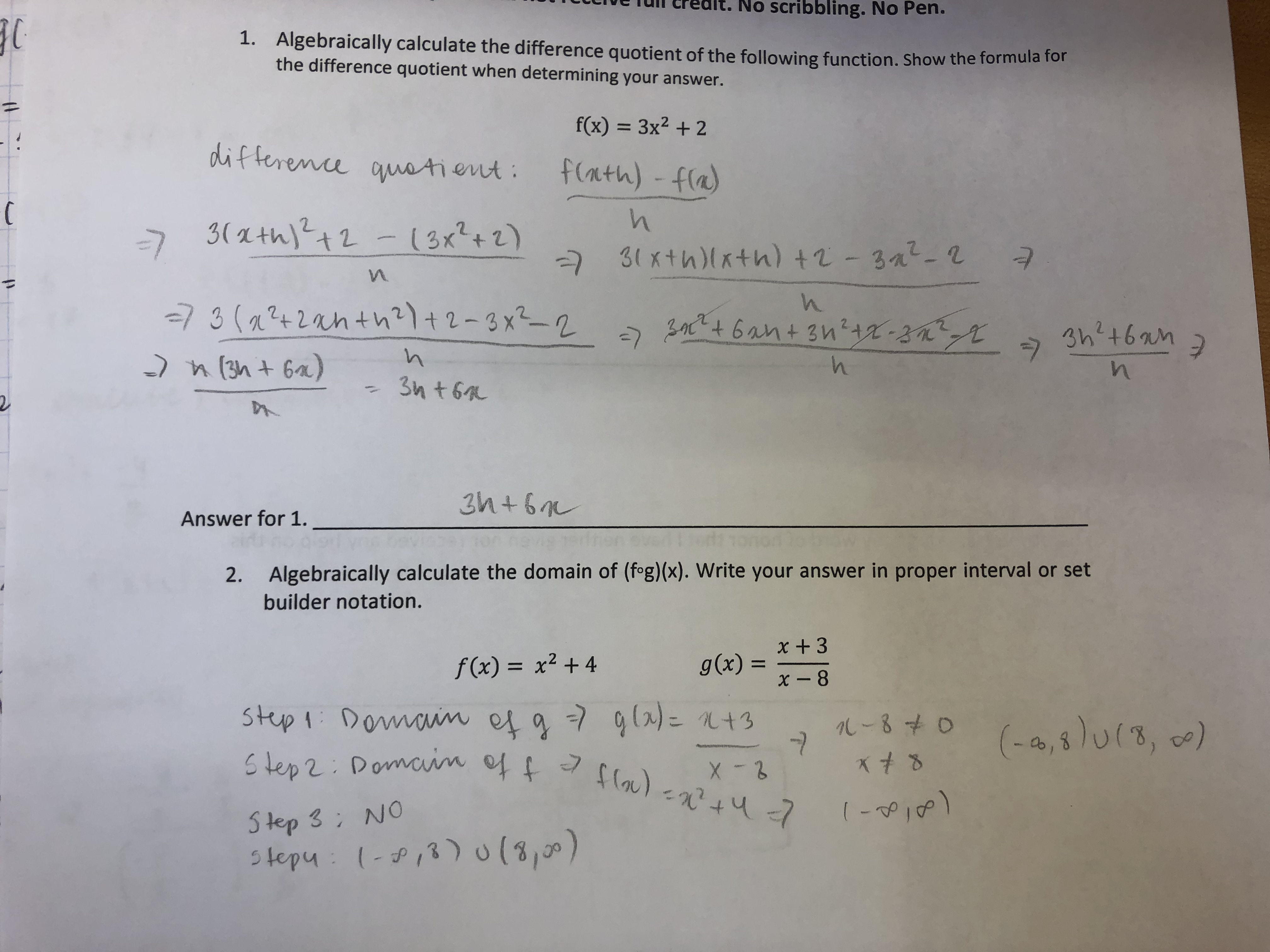# tueueredlt. No scribbling. No Pen. Algebraically calculate the difference quotient of the following function. Show the form the difference quotient when determining your answer. 1. ula for fx)3x2 +2 ent: 5111 Answer for 1. Algebraically calculate the domain of (fog)(x). Write your answer in proper interval or set builder notation. 2. x +3 f(x) = x2 + 4 gr)8 244 tepy

Question

Can you check my question 2? Please. Thank you so muchhelp_outlineImage Transcriptionclosetueueredlt. No scribbling. No Pen. Algebraically calculate the difference quotient of the following function. Show the form the difference quotient when determining your answer. 1. ula for fx)3x2 +2 ent: 5111 Answer for 1. Algebraically calculate the domain of (fog)(x). Write your answer in proper interval or set builder notation. 2. x +3 f(x) = x2 + 4 gr)8 244 tepy fullscreen